# KSEEB SSLC Class 10 Maths Solutions Chapter 11 Introduction to Trigonometry Ex 11.1

KSEEB SSLC Class 10 Maths Solutions Chapter 11 Introduction to Trigonometry Ex 11.1 are part of KSEEB SSLC Class 10 Maths Solutions. Here we have given Karnataka SSLC Class 10 Maths Solutions Chapter 11 Introduction to Trigonometry Exercise 11.1.

## Karnataka SSLC Class 10 Maths Solutions Chapter 11 Introduction to Trigonometry Exercise 11.1

Question 1.
In ∆ABC, right-angled at B, AB = 24 cm., BC = 7 cm. Determine:
i) sin A, cos A
ii) sin C, cos C
Solution: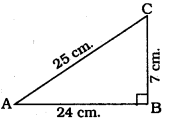In ⊥∆ABC, ∠B = 90°
As per Pythagoras theorem
AC2 = AB2 + BC2
= (24)2 + (7) 2
= 576 + 49
AC2 = 625
∴ AC = 25 cmQuestion 2.
In the given figure, find tan P – cot R.
Solution:In ⊥∆PQR, ∠Q = 90°
∴ PQ2 + QR2 = PR2
(12)2 + QR2 = (13)2
144 + QR2 = 169
QR2 = 169 – 144
QR2 = 25
∴ QR = 5 cm.Question 3.
If sin A = $$\frac{3}{4}$$ calculate cos A and tan A.
Solution: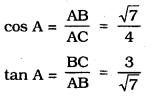Question 4.
Given 15 cot A = 8, find sin A and sec A.
Solution: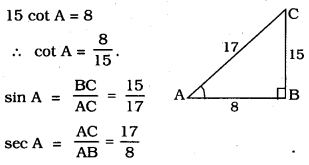Question 5.
Given sec θ = $$\frac{13}{12}$$, calculate all other trigonometric ratios
Solution;Question 6.
If ∠A and ∠B are acute angles such that cos A = cos B, then show that ∠A = ∠B.
Solution:
In ⊥∆ABC, ∠A and ∠B are acute angles. CD ⊥ AB is drawn.CD is common.
S.S.S. Postulate :
∴ ∠A = ∠B
∵ “Angles of similar triangles are equiangular.”

Question 7.
If cot θ = $$\frac{7}{8}$$, evaluate :
(i) $$\frac{(1+\sin \theta)(1-\sin \theta)}{(1+\cos \theta)(1-\cos \theta)}$$
(ii) cot2θ
Solution:Question 8.
If 3 cot A = 4, check whether $$\frac{1-\tan ^{2} A}{1+\tan ^{2} A}$$ = cos2 A – sin2 A or not
Solution: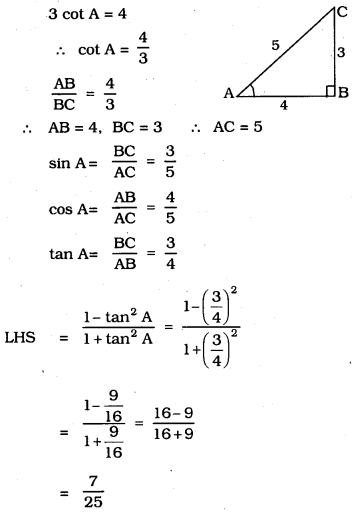Question 9.
In ∆ABC, right-angled at B, if tan A =$$\frac{1}{\sqrt{3}}$$ find the value of:
i) sin A cos C + cos A sin C
ii) cos A cos C – sin A sin C
Solution: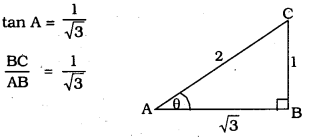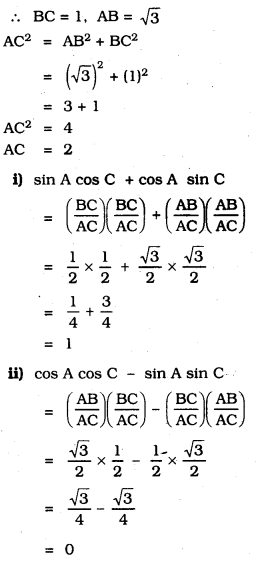Question 10.
In ∆PQR, right-angled at Q, PR + QR = 25 cm. and PQ = 5 cm. Determine the values of sin P, cos P and tan P
Solution:
PQ = 5 cm
PR + QR = 25 cm
∴ PR = 25 – QRPR2 = PQ2 + QR2
QR2 = PR2 – PQ2
= (25 – QR)2 – (5)2
QR2 = 625 – 50QR + QR2 – 25
50QR = 600
∴ QR = 12 cm.
∴ PR = 25 – QR = 25 – 12 = 13 cm.
∴ QR = 12 cm
∴ PR = 25 – QR = 25 – 12 = 13 cm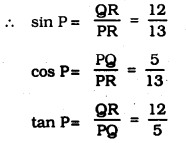Question 11.
(i) The value of tan A is always less than 1.
False. 60° = $$\sqrt{3}$$ > 1

(ii) If sec A = $$\frac{12}{5}$$ for some value of angle A.
True. because sec A > 1.

(iii) cos A is the abbreviation used for the cosecant of angle A.
False. Because cos A is simplified as cos.

(iv) cot A is the product of cot and A.
cot A = $$\frac{\text { Adjacent side }}{\text { Opposite side }}$$
(v) sin θ = $$\frac{4}{3}$$ for some angle θ